Students can download Maths Chapter 3 Algebra Ex 3.14 Questions and Answers, Notes, Samacheer Kalvi 10th Maths Guide Pdf helps you to revise the complete Tamilnadu State Board New Syllabus, helps students complete homework assignments and to score high marks in board exams.

## Tamilnadu Samacheer Kalvi 10th Maths Solutions Chapter 3 Algebra Ex 3.14

Question 1.
Write each of the following expression in terms of α + β and αβ
(i) $$\frac{\alpha}{3 \beta}+\frac{\beta}{3 \alpha}$$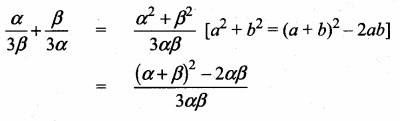(ii) $$\frac{1}{\alpha^{2} \beta}+\frac{1}{\beta^{2} \alpha}$$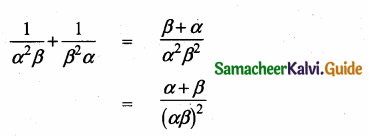(iii) (3α – 1) (3β – 1)
(3α – 1) (3β – 1) = 9αc – 3α – 3β + 1
= 9αβ – 3(α + β) + 1(iv) $$\frac{\alpha+3}{\beta}+\frac{\beta+3}{\alpha}$$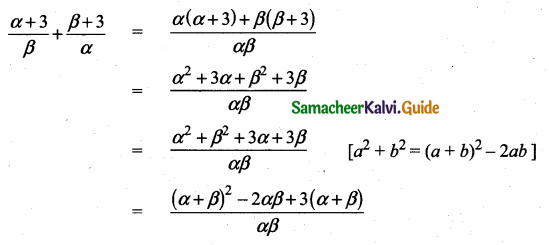Question 2.
The roots of the equation 2x2 – 7x + 5 = 0 are a and p. Find the value of [without solving the equation]
$$\text { (i) } \frac{1}{\alpha}+\frac{1}{\beta}$$
α and α are the roots of the equation 2x2 – 7x + 5 = 0
α + β = $$\frac { 7 }{ 2 }$$ ; αβ = $$\frac { 5 }{ 2 }$$
(i) $$\frac{1}{\alpha}+\frac{1}{\beta}$$ = $$\frac{\beta+\alpha}{\alpha \beta}$$
= $$\frac { 7 }{ 2 }$$ + $$\frac { 5 }{ 2 }$$ = $$\frac { 7 }{ 2 }$$ × $$\frac { 2 }{ 5 }$$ = $$\frac { 7 }{ 5 }$$

(ii) $$\frac{\alpha}{\beta}+\frac{\beta}{\alpha}$$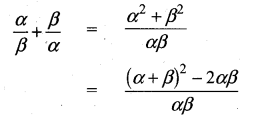= ($$\frac { 7 }{ 2 }$$)2 – 2 × $$\frac { 5 }{ 2 }$$ ÷ $$\frac { 5 }{ 2 }$$
= $$\frac { 49 }{ 4 }$$ – 5 ÷ $$\frac { 5 }{ 2 }$$ = $$\frac { 49-20 }{ 4 }$$ ÷ $$\frac { 5 }{ 2 }$$
= $$\frac { 29 }{ 4 }$$ × $$\frac { 2 }{ 5 }$$ = $$\frac { 29 }{ 10 }$$(iii) $$\frac{\alpha+2}{\beta+2}+\frac{\beta+2}{\alpha+2}$$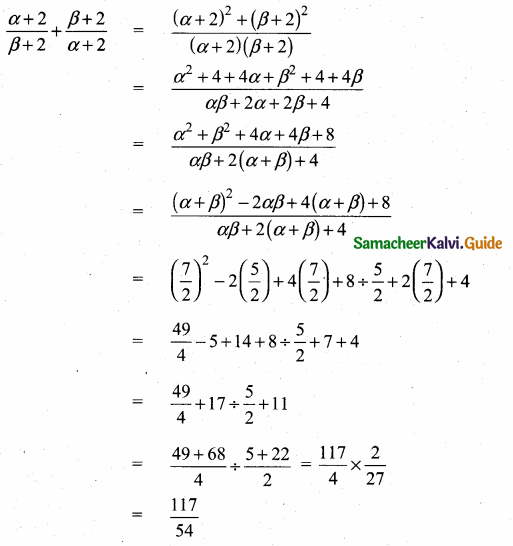Question 3.
The roots of the equation x2 + 6x – 4 = 0 are a, p. Find the quadratic equation whose roots are
(i) α2 and β2
α and β are the roots of x2 + 6x – 4 = 0
α + β = -6; αβ = -4

(i) Sum of the roots = α2 + β2
= (α + β)2 – 2αβ
= 36 – 2 – (4) = 36 + 8
= 44
Product of the roots = α2 + β2
= (αβ)2
= (-4)2
= 16
x2 – (sum of the roots) x + Product of the roots = 0
x2 – (44)x + 16 = 0
x2 – 44x + 16 = 0

(ii) $$\frac{2}{\alpha}$$ and $$\frac{2}{\beta}$$
Sum of the roots = $$\frac{2}{\alpha}$$ + $$\frac{2}{\beta}$$
= $$\frac{2 \beta+2 \alpha}{\alpha \beta}=\frac{2(\alpha+\beta)}{\alpha \beta}$$
= $$\frac{2(-6)}{-4}=\frac{-12}{-4}=3$$
Product of the roots = $$\frac{2}{\alpha} \times \frac{2}{\beta}=\frac{4}{\alpha \beta}$$
= $$\frac { 4 }{ -4 }$$ = -1
x2 – (sum of the roots) x + Product of the roots = 0
x2 – 3x – 1 = 0

(iii) α2β and β2α
Sum of the roots = α2β + β2α
= αβ (α + β)
= -4 (-6) = 24
Product of the roots = α2β × β2α
= α2β3 = (αβ)3
= (-4)3 = -64
x2 – (Sum of the roots) x + Product of the roots = 0
x2 – 24x – 64 = 0Question 4.
If α, β are the roots of 7x2 + ax + 2 = 0 and if β – α = $$\frac { 13 }{ 7 }$$ Find the values of a.
α and β are the roots of 7x2 + ax + 2 = 0
α + β = $$\frac { -a }{ 7 }$$; αβ = $$\frac { 2 }{ 7 }$$
Given β – α = – $$\frac { 13 }{ 7 }$$ ⇒ α – β = $$\frac { 13 }{ 7 }$$
Squaring on both sides
(α – β)2 = ($$\frac { 13 }{ 7 }$$)2
α2 + β2 = 2αβ = $$\frac { 169 }{ 49 }$$
(- $$\frac { a }{ 7 }$$)2 -4($$\frac { 2 }{ 7 }$$) = $$\frac { 169 }{ 49 }$$ ⇒ $$\frac{a^{2}}{49}-\frac{8}{7}=\frac{169}{49}$$
$$\frac{a^{2}}{49}$$ = $$\frac { 225 }{ 49 }$$ ⇒ a2 = $$\frac{225 \times 49}{49}$$
a2 = 225 ⇒ a = ± $$\sqrt { 225 }$$ = ± 15
The value of a = 15 or – 15

Question 5.
If one root of the equation 2y2, – ay + 64 = 0 is twice the other then find the values of a.
Let the roots be α and 2α
Here a = 2, b = – a, c = 64
Sum of the roots = – $$\frac { b }{ a }$$
α + 2α = $$\frac { a }{ 2 }$$
3α = $$\frac { a }{ 2 }$$
a = 6α …….(1)
Product of the roots = $$\frac { c }{ a }$$
α × 2α = $$\frac { 64 }{ 2 }$$ = 2α2 = 32
α2 = $$\frac { 32 }{ 2 }$$ = 16
α = $$\sqrt { 16 }$$ = ± 4
Substitute the value of a in (1)
When α = 4
a = 6(4)
a = 24
The Value of a is 24 or -24
When α = -4
a = 6(-4)
a = -24Question 6.
If one root of the equation 3x2 + kx + 81 = 0 (having real roots) is the square of the other then find k.
Let α and α2 be the root of the equation 3x2 + kx + 81
Here a = 3, b = k, c = 81
Sum of the roots = – $$\frac { b }{ a }$$ = – $$\frac { k }{ 3 }$$
α + α2 = –$$\frac { k }{ 3 }$$
3α + 3α2 = -k ……..(1)
Product of the roots = $$\frac { c }{ a }$$ = $$\frac { 81 }{ 3 }$$ = 27
α × α2 = 27
α3 = 27 ⇒ α3 = 33
α = 3
Substitute the value of α = 3 in (1)
3(3) + 3(3)2 = -k
9 + 27 = -k ⇒ 36 = – k
∴ k = -36
The value of k = -36